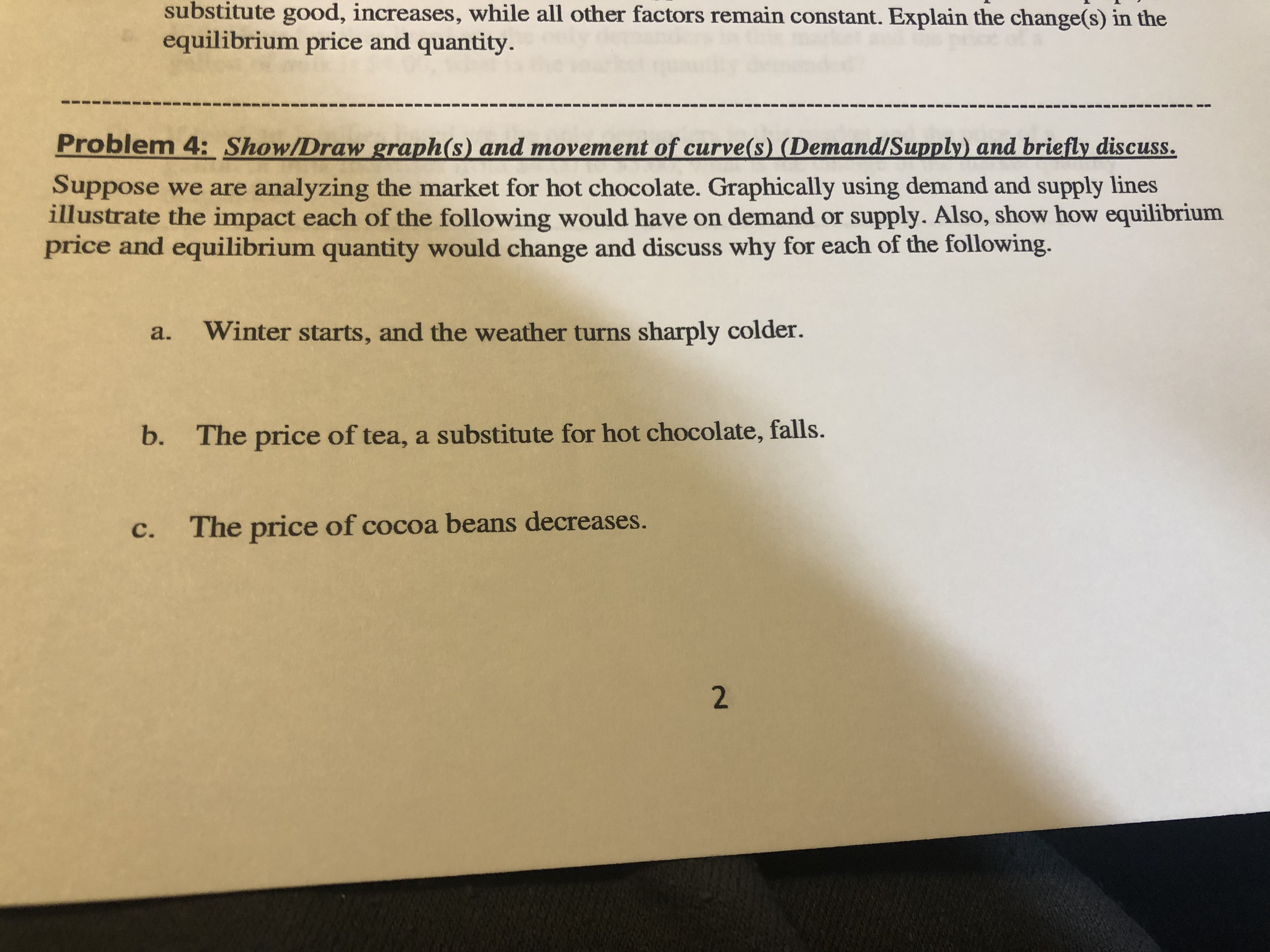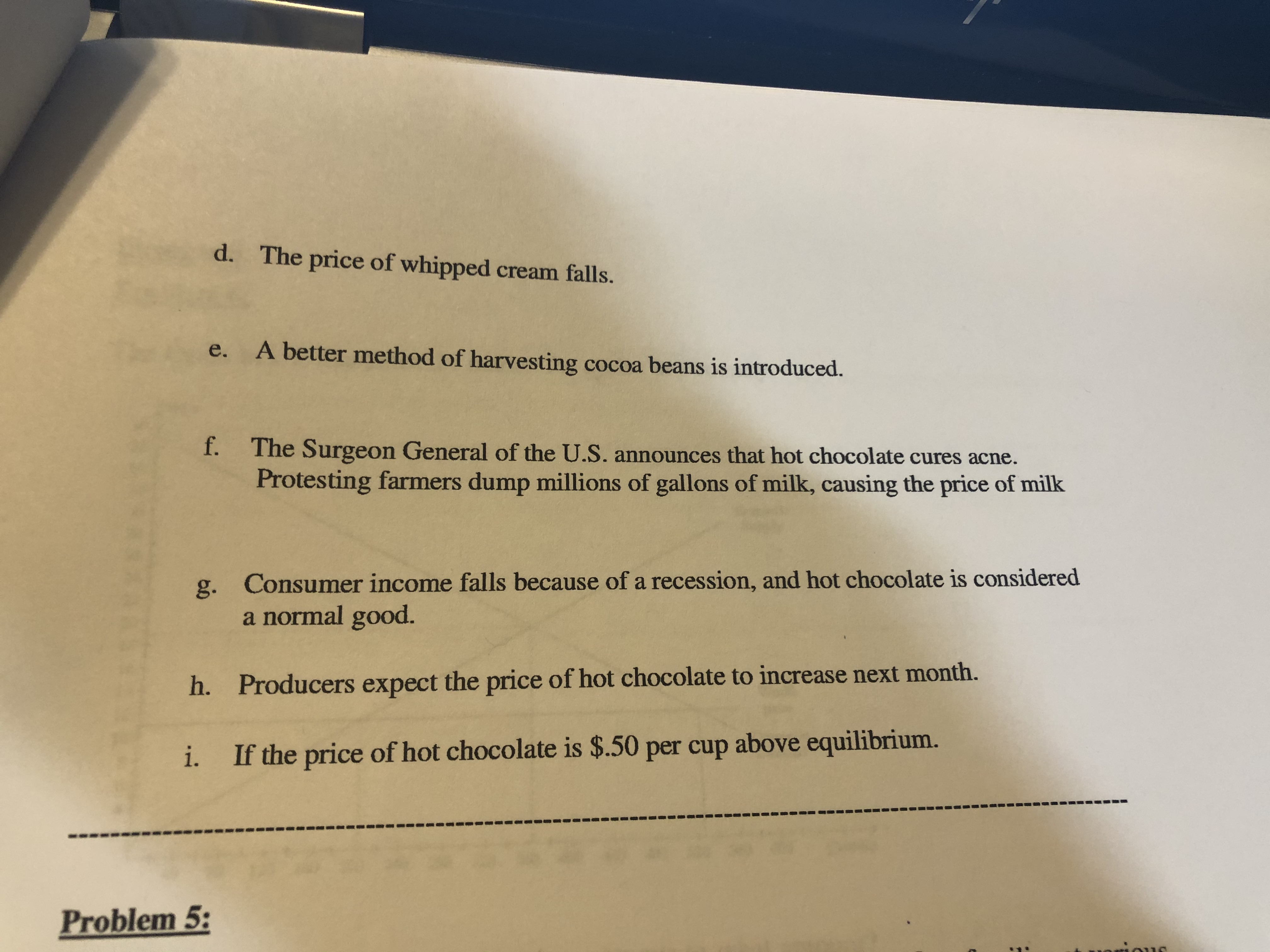# substitute good, increases, while all other factors remain constant. Explain the change(s) in theequilibrium price and quantityProblem 4: Show/Draw graph(s) and movement of curve (s) (Demand/Supply) and briefly discuss.Suppose we are analyzing the market for hot chocolate. Graphically using demand and supply linesillustrate the impact each of the following would have on demand or supply. Also, show how equilibriumprice and equilibrium quantity would change and discuss why for each of the following.Winter starts, and the weather turns sharply colder.a.b.The price of tea, a substitute for hot chocolate, falls.The price of cocoa beans decreases.с.2 d.The price of whipped cream falls.A better method of harvesting cocoa beans is introduced.e.f.The Surgeon General of the U.S. announces that hot chocolate cures acne.Protesting farmers dump millions of gallons of milk, causing the price of milkConsumer income falls because of a recession, and hot chocolate is considereda normal good.g.Producers expect the price of hot chocolate to increase next month.h.If the price of hot chocolate is \$.50 per cup above equilibrium.i.Problem 5:

Question
11 viewshelp_outlineImage Transcriptionclosesubstitute good, increases, while all other factors remain constant. Explain the change(s) in the equilibrium price and quantity Problem 4: Show/Draw graph(s) and movement of curve (s) (Demand/Supply) and briefly discuss. Suppose we are analyzing the market for hot chocolate. Graphically using demand and supply lines illustrate the impact each of the following would have on demand or supply. Also, show how equilibrium price and equilibrium quantity would change and discuss why for each of the following. Winter starts, and the weather turns sharply colder. a. b. The price of tea, a substitute for hot chocolate, falls. The price of cocoa beans decreases. с. 2 fullscreenhelp_outlineImage Transcriptionclosed. The price of whipped cream falls. A better method of harvesting cocoa beans is introduced. e. f. The Surgeon General of the U.S. announces that hot chocolate cures acne. Protesting farmers dump millions of gallons of milk, causing the price of milk Consumer income falls because of a recession, and hot chocolate is considered a normal good. g. Producers expect the price of hot chocolate to increase next month. h. If the price of hot chocolate is \$.50 per cup above equilibrium. i. Problem 5: fullscreen
check_circle

Step 1

Since we only answer up to 3 sub-parts, we’ll answer the first 3.

Please resubmit the question and specify the other subparts (up to 3) you’d like answered.

Step 2

a) With reference to the graph below, if the economy is in equilibrium initially, the supply and demand intersect each other at pt. E. There is equilibrium price and quantity at this point. Now, if sudden winters turn the weather cold, the demand for hot chocolate will increase. It will shift demand curve to right. Due to this, the economy reaches at point F. There is an excess demand at this point. So, to reach the equilibrium point, the prices in the economy rises to P’. Hence, the new equilibrium is achieved at point E’ with new price P’ and new equilibrium quantity Q’.

Step 3

b) With reference to the graph below, if the economy is in equilibrium initially, the supply and demand intersect each other at pt. E. There is equilibrium price and quantity at this point. Now, if the price of tea decreases, its quantity demanded will increase. As tea and hot chocolate are...

### Want to see the full answer?

See Solution

#### Want to see this answer and more?

Solutions are written by subject experts who are available 24/7. Questions are typically answered within 1 hour.*

See Solution
*Response times may vary by subject and question.
Tagged in